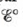Chapter 18, Problem 49E

Chapter
Section
Textbook Problem

# Chlorine dioxide (C1O2), which is produced by the reaction 2 NaC1O 2 ( a q )   +  Cl 2 ( g ) → 2ClO 2 ( g ) +  2NaCl ( a q ) has been tested as a disinfectant for municipal water treatment. Using data from Table 17-1, calculateand ∆G° at 25°C for the production of ClO2.

Interpretation Introduction

Interpretation:

The reaction involved in the production of chlorine dioxide (ClO2) is given. The value of E° and ΔG° for the given chemical reaction is to be calculated.

Concept introduction:

The species with higher reduction potential undergoes reduction at the cathode while the species with lower reduction potential undergoes oxidation at the anode. The change in Gibbs free energy is the useful amount work that can be done and negative value of ΔG° shows that the reaction is spontaneous in nature.

To determine: The value of E° and ΔG° for the given reaction.

Explanation

The value of E° is calculated as 0.41V_ .

The reaction taking place at cathode is,

Cl2(g)+2e2Cl(aq)E°red=1.36V

The reaction taking place at anode is,

2ClO2(aq)2ClO2(aq)+2eE°ox=0.954V

Add both the oxidation and reduction half-reaction,

2ClO2(aq)+Cl2(g)2ClO2(aq)+2Cl(aq)

The overall cell potential is calculated as,

E°cell=E°ox+E°red=0.954V+1.36V=0.406V0.41V_

The value of E°cell is 0.41V_

### Still sussing out bartleby?

Check out a sample textbook solution.

See a sample solution

#### The Solution to Your Study Problems

Bartleby provides explanations to thousands of textbook problems written by our experts, many with advanced degrees!

Get Started